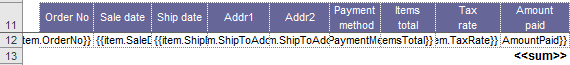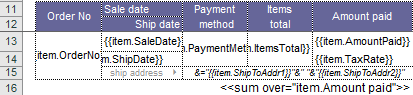View on GitHub# Totals in a Column

In order to get the totals for the column in ClosedXML.Report there are aggregation tags:

• SUM - displays the amount of the column;
• AVG or AVERAGE - the average value of the column;
• COUNT - the number of values in a column;
• COUNTNUMS - the number of non-empty values in the column;
• MAX - the maximum value in the column;
• MIN - the minimum value in the column;
• PRODUCT - product by column;
• STDEV - standard deviation;
• STDEVP - standard deviation of the total population
• VAR - dispersion;
• VARP - dispersion for the general population.

To calculate the results of ClosedXML.Report uses Excel tools, i.e. Each of these tags will be replaced by the corresponding Excel formula. For example, to calculate the Amount paid amount, we need to add the `<<sum>>` tag to the options line.Each aggregation tag has a `over` parameter providing you with a powerful tool that allows you to perform more complex calculations that Excel cannot do for various reasons. In particular, Excel will not be able to calculate the amount of a complex (multi-line) area. The argument to over is an expression. Example:``````<<sum over="item.AmountPaid">>
``````

This function is very useful for computing subtotals in master-detail reports. You can see an example in the Report examples section